﻿ 一种基于轨迹预测的机会网络路由协议
«上一篇文章快速检索 高级检索

 应用科技2020, Vol. 47Issue (3): 94-99  DOI: 10.11991/yykj.2019090200

### 引用本文WANG Tong, SHAN Xin, ZHENG Xinrui. An opportunistic network routing protocol based on trajectory prediction[J]. Applied Science and Technology, 2020, 47(3): 94-99. DOI: 10.11991/yykj.201909020.### 文章历史

1. 哈尔滨工程大学 信息与通信工程学院，黑龙江 哈尔滨 150001;
2. 加拿大多伦多大学 机械与工业工程系，安大略 多伦多 M5S 1A1

An opportunistic network routing protocol based on trajectory prediction
WANG Tong1, SHAN Xin1, ZHENG Xinrui2
1. College of Information and Communication Engineering, Harbin Engineering University, Harbin 150001, China;
2. Department of Mechanical and Industrial Engineering, University of Toronto, Toronto M5S 1A1, Canada
Abstract: According to the characteristics such as frequent disconnection of links, the high-speed movement of nodes and the density of sparse network in opportunistic networks (ONs), this paper proposes a method based on the historical data of node movement and the nodes’ sociality to predict trajectory of nodes, and then to select relay nodes to complete the routing protocol of the opportunity network for message transmission. First, conditional entropy is used to analyze the predictability of node trajectories. Then, the node’s unit active area is divided according to the node’s speed and prediction unit. Finally, using the node movement probability and its sociality, the next position of the node is predicted. The simulation results show that the trajectory prediction can effectively solve the problem that the network connectivity is easily disconnected due to the high-speed movement of the nodes and the constant change of nodes’ position. The communication protocol based on trajectory prediction has a good delivery success rate and achieves high-efficiecy delivery of messages.
Keywords: opportunity network    trajectory prediction    historical data    conditional entropy    meshing    sociality    probability calculation    message delivery

1 轨迹分析表 1 节点轨迹分析的符号定义

 $P\left( {{L_m}} \right) = \frac{{{n_m}}}{{{n_0}}}$
 $H\left( L \right) = - \sum\limits_{m = 1}^n {P\left( {{L_m}} \right) \cdot {{{\rm{lb}} }}} P\left( {{L_m}} \right)$

 $P\left( {{L_m},{L_{m - k}}} \right) = \frac{{{n_{m - 1,m}}}}{{{n_1}}}$
 $\begin{array}{l} H\left( {L|{L_{m - 1}}} \right) = H\left( {L,{L_{m - 1}}} \right) - H\left( {{L_{m - 1}}} \right) = \\ - \displaystyle\sum\limits_{m = 1}^n {P\left( {{L_m},{L_{m - 1}}} \right) \cdot {{{\rm{lb}} }}} P\left( {{L_m},{L_{m - 1}}} \right) + \\ \displaystyle\sum\limits_{m = 1}^n {P\left( {{L_{m - 1}}} \right) \cdot {{{\rm{lb}} }}} P\left( {{L_{m - 1}}} \right) \\ \end{array}$Download: 图 1 轨迹分析仿真

2 轨迹预测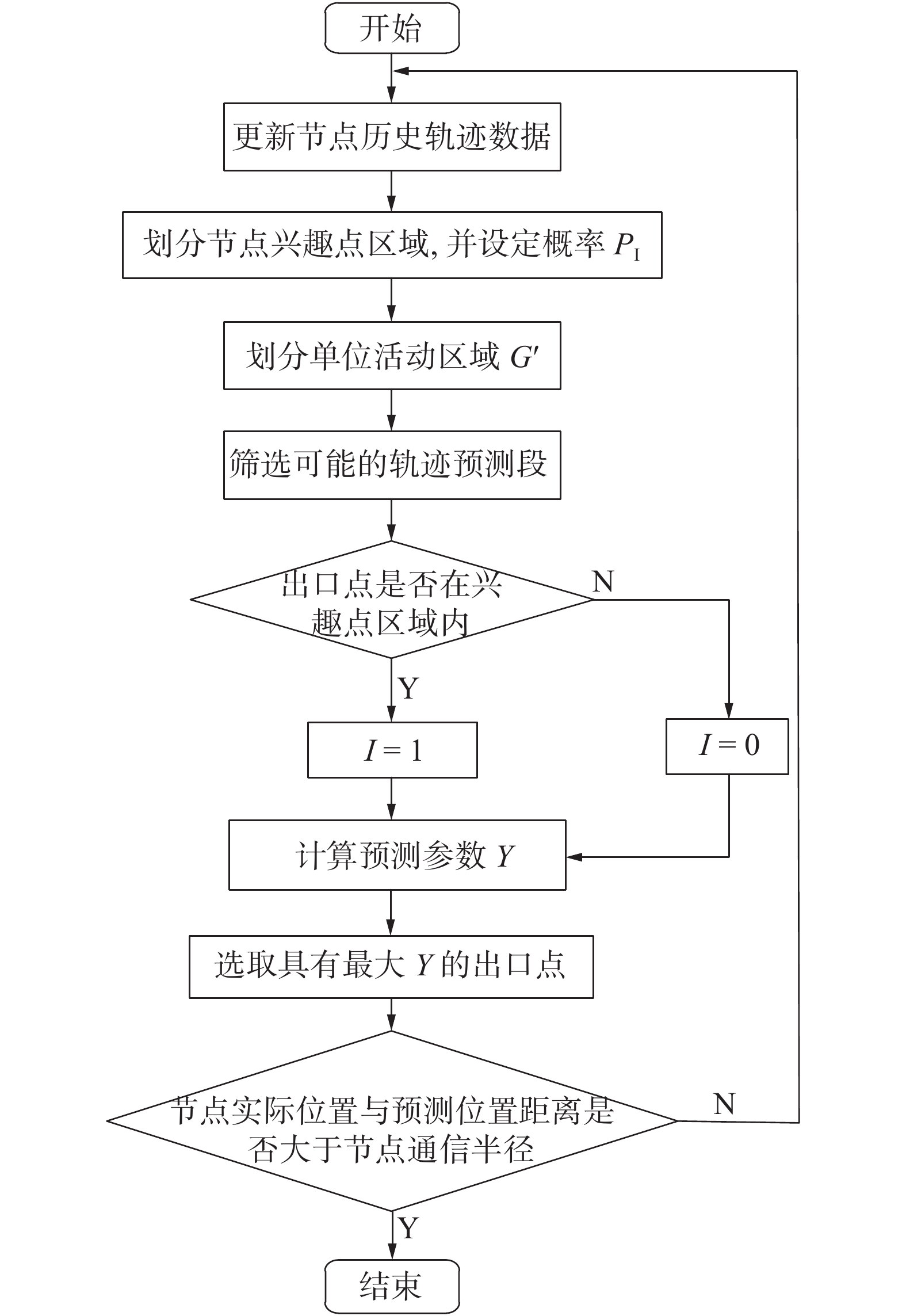Download: 图 2 轨迹预测流程
2.1 单位活动区域划分

 $\begin{split} T =& \left\{ {\left[ {\left( {{s_0},{c_0}} \right),\left( {{v_0},{t_0}} \right)} \right], \cdots ,} \right.\left[ {\left( {{s_i},{c_i}} \right),\left( {{v_i},{t_i}} \right)} \right], \cdots ,\\ &\left[ {\left( {{s_{N - 1}},{c_{N - 1}}} \right),} \right. \left. {\left. {\left( {{v_{N - 1}},{t_{N - 1}}} \right)} \right]} \right\} \end{split}$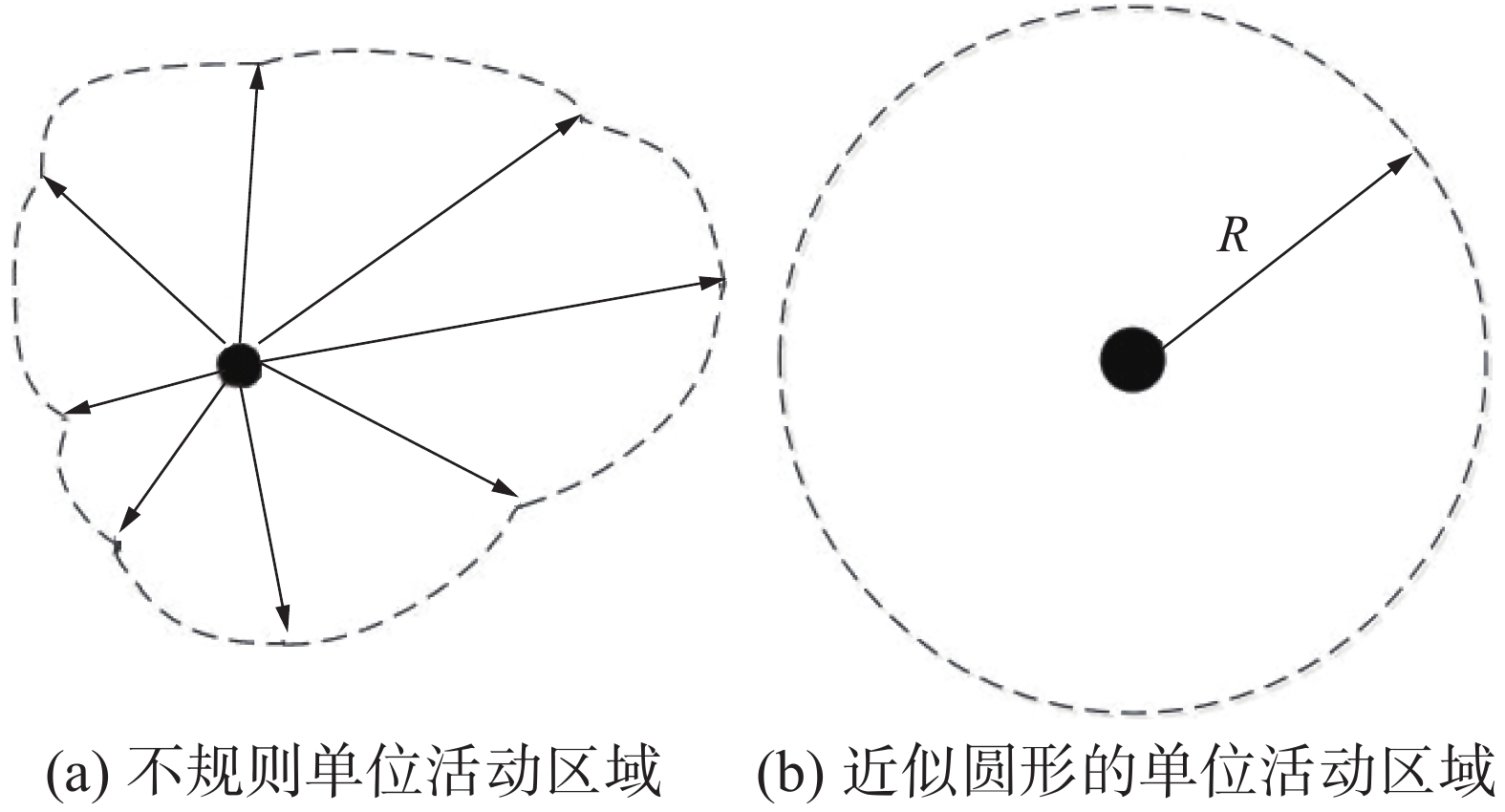Download: 图 3 单位活动区域
 $R=\Delta D=D_{n}-D_{n-1}=\left(\frac{v_{n}+v_{n-1}}{2}\right) \cdot \Delta t$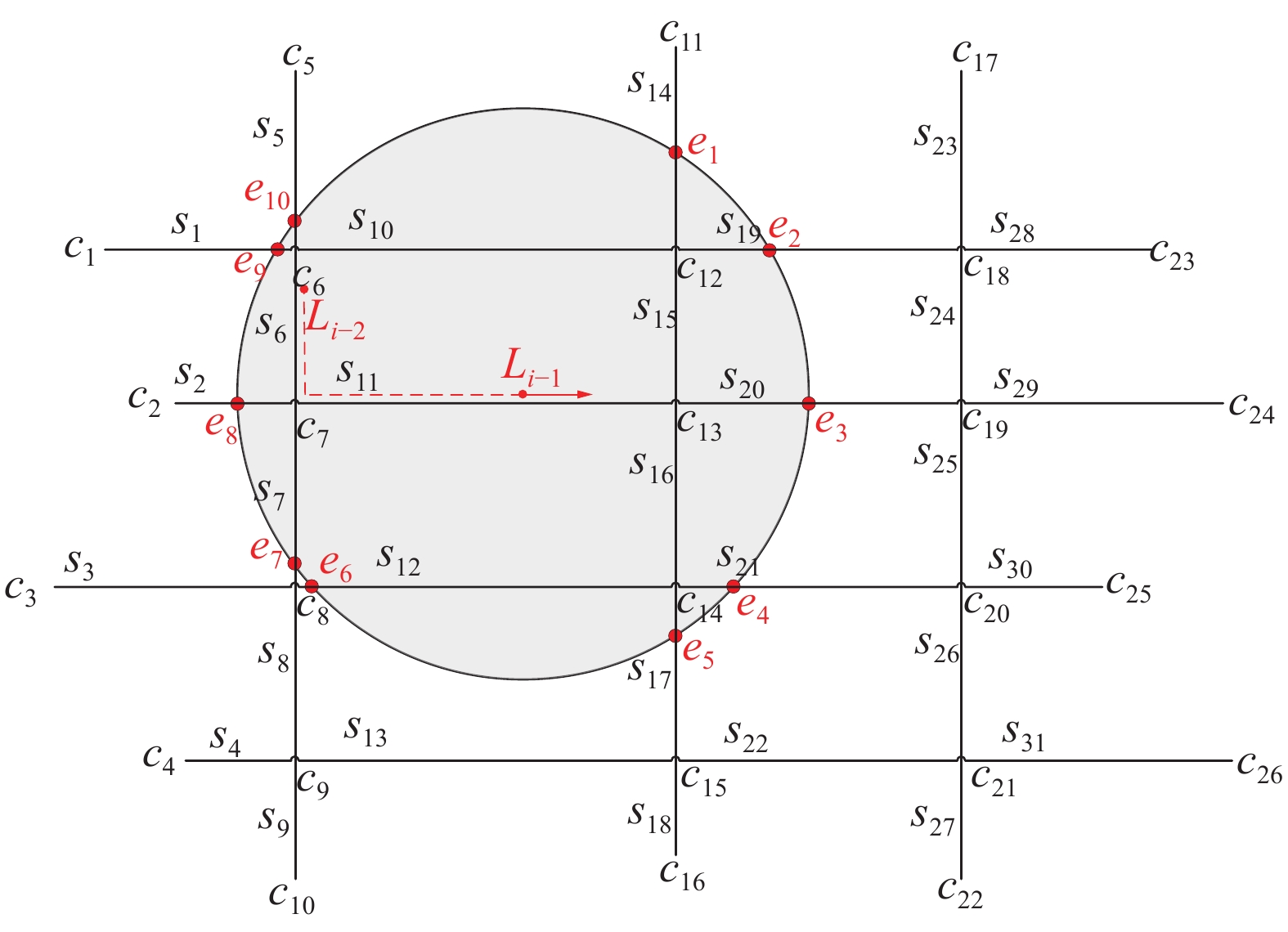Download: 图 4 模拟城市道路网络
2.2 路径选择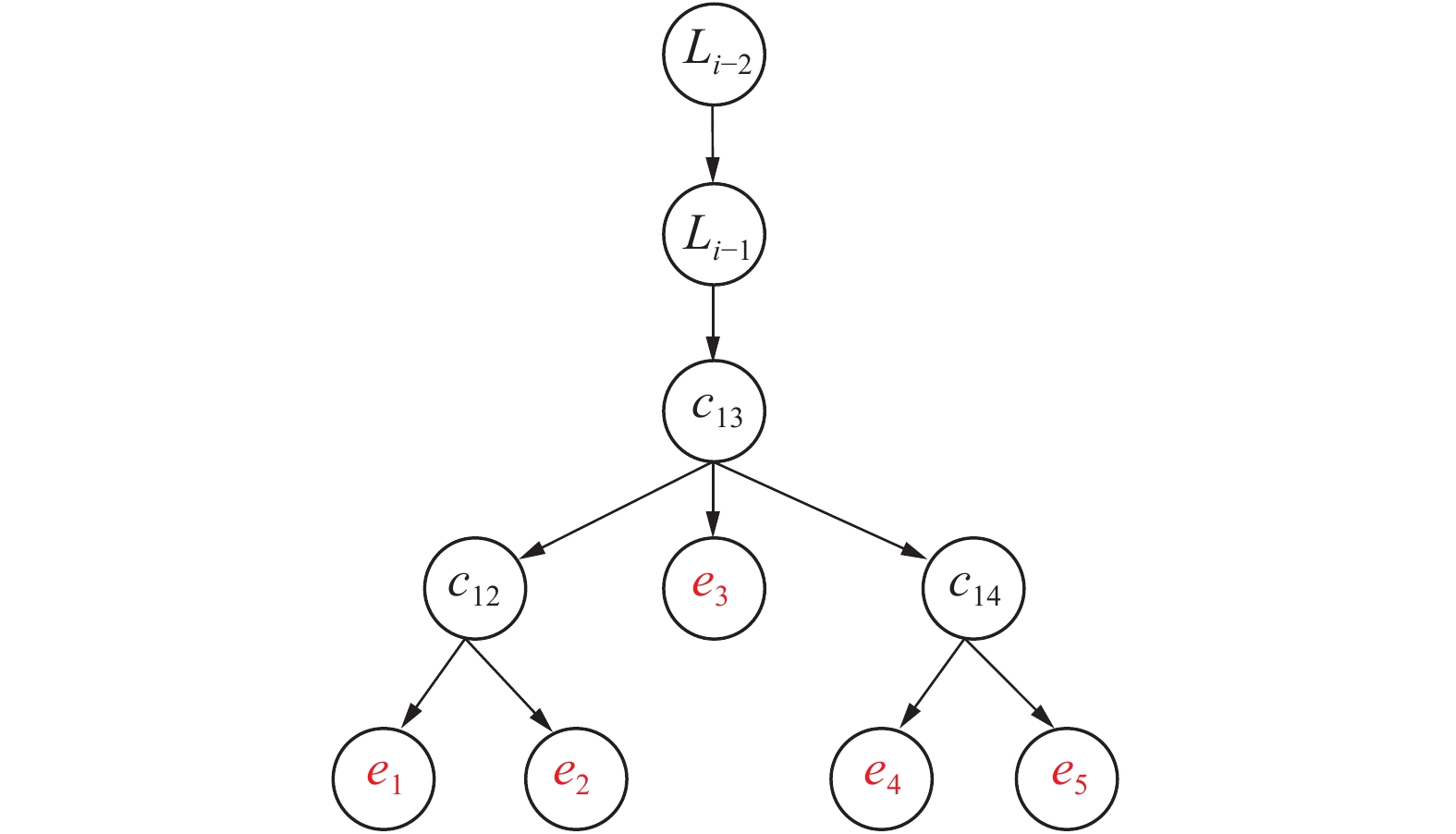Download: 图 5 轨迹预测段的可能情况
2.3 概率计算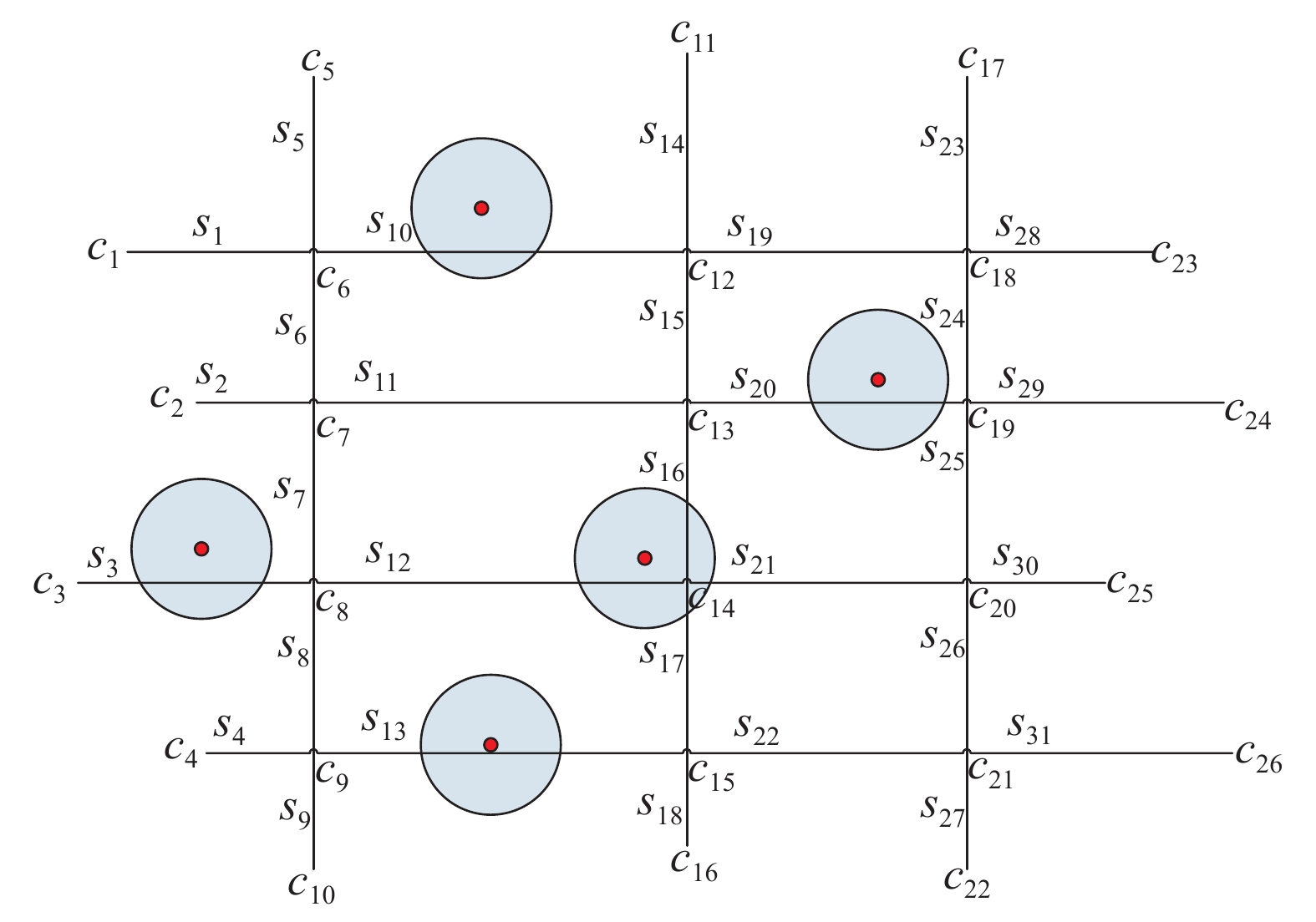Download: 图 6 兴趣点区域分布

 $I = \left\{ \begin{array}{l} 0,{\rm{ }}{\text{出口点不在兴趣点区域}}{\rm{ }}\\ 1,{\rm{ }}{\text{出口点落入兴趣点区域}} \end{array} \right.$

 ${{M}}\left(S, t_{N}\right)=\left[\begin{array}{cccc} P\left(S_{0} | {S}_{0}\right) & P\left(S_{1} | {S}_{0}\right) & \cdots & P\left(S_{n} | {S}_{0}\right) \\ P\left(S_{0} | {S}_{1}\right) & P\left(S_{1} | {S}_{1}\right) & \cdots & P\left(S_{n} | {S}_{1}\right) \\ \vdots & \vdots & & \vdots \\ P\left(S_{0} | {S}_{n}\right) & P\left(S_{1} | {S}_{n}\right) & \cdots & P\left(S_{n} | {S}_{n}\right) \end{array}\right]$

 $P\left( {{S_e}|{S_c}} \right) = \frac{{{U_{0,n}}}}{{{U_0}}}$

 $Y = \frac{1}{3}I \cdot {P_I} + \frac{2}{3}P\left( {{S_e}|{S_c}} \right)$

3 仿真结果及分析 3.1 仿真环境表 2 仿真参数设置
3.2 结果分析

3.2.1 节点数量对算法性能的影响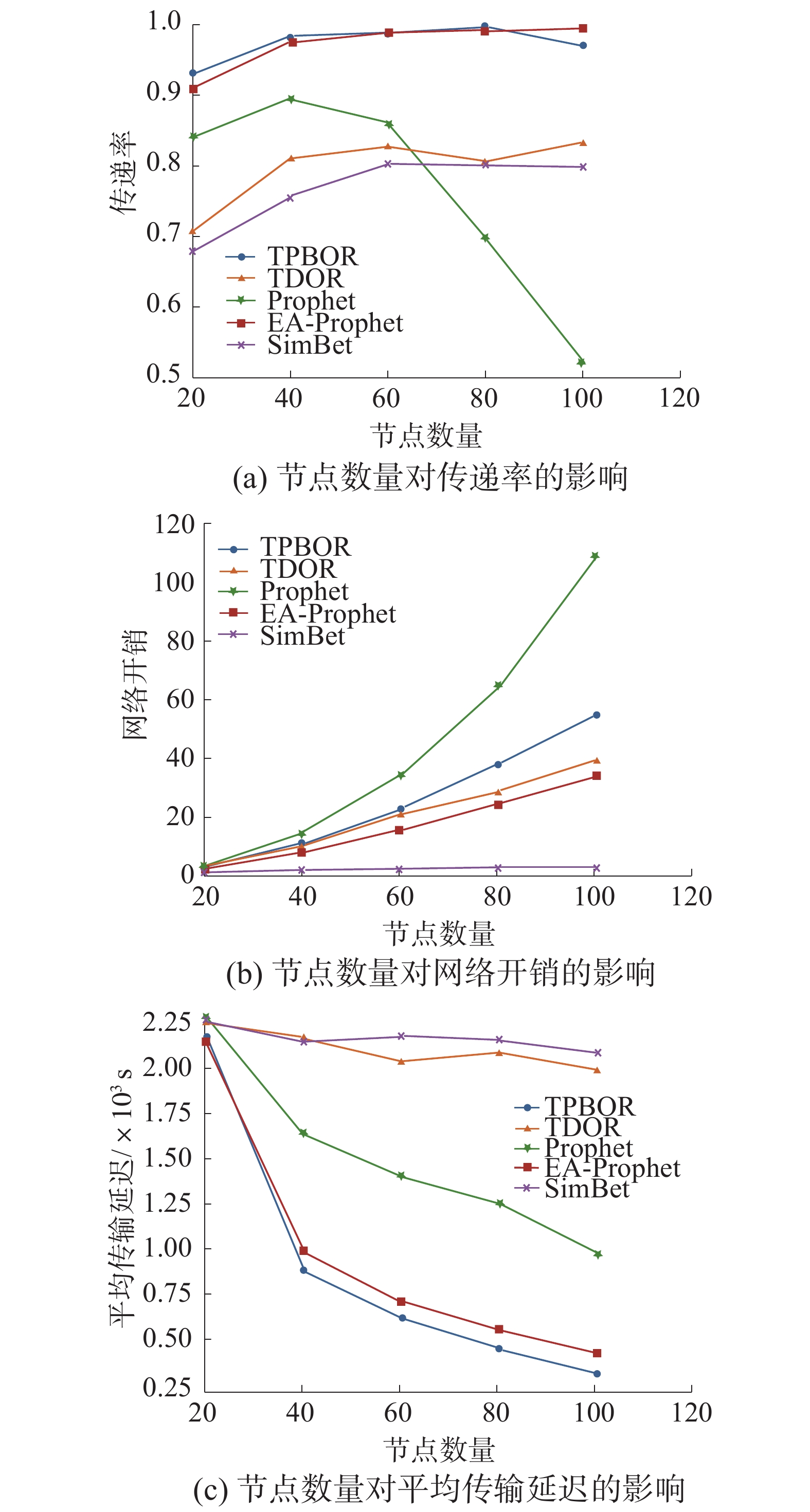Download: 图 7 节点数量对路由协议的影响

3.2.2 节点缓存大小对算法性能的影响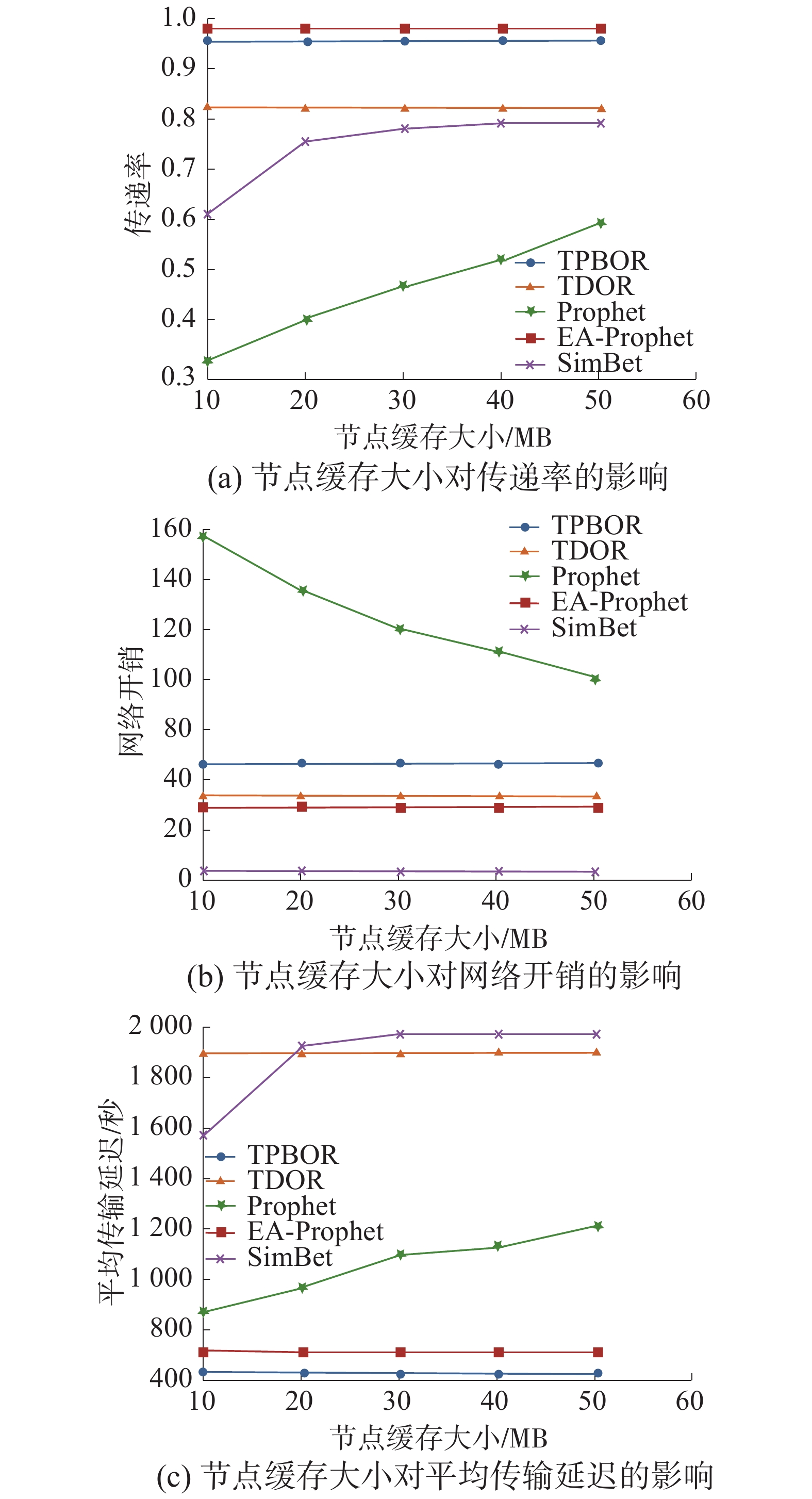Download: 图 8 节点缓存对路由协议的影响

3.2.3 不同消息生存周期对算法性能的影响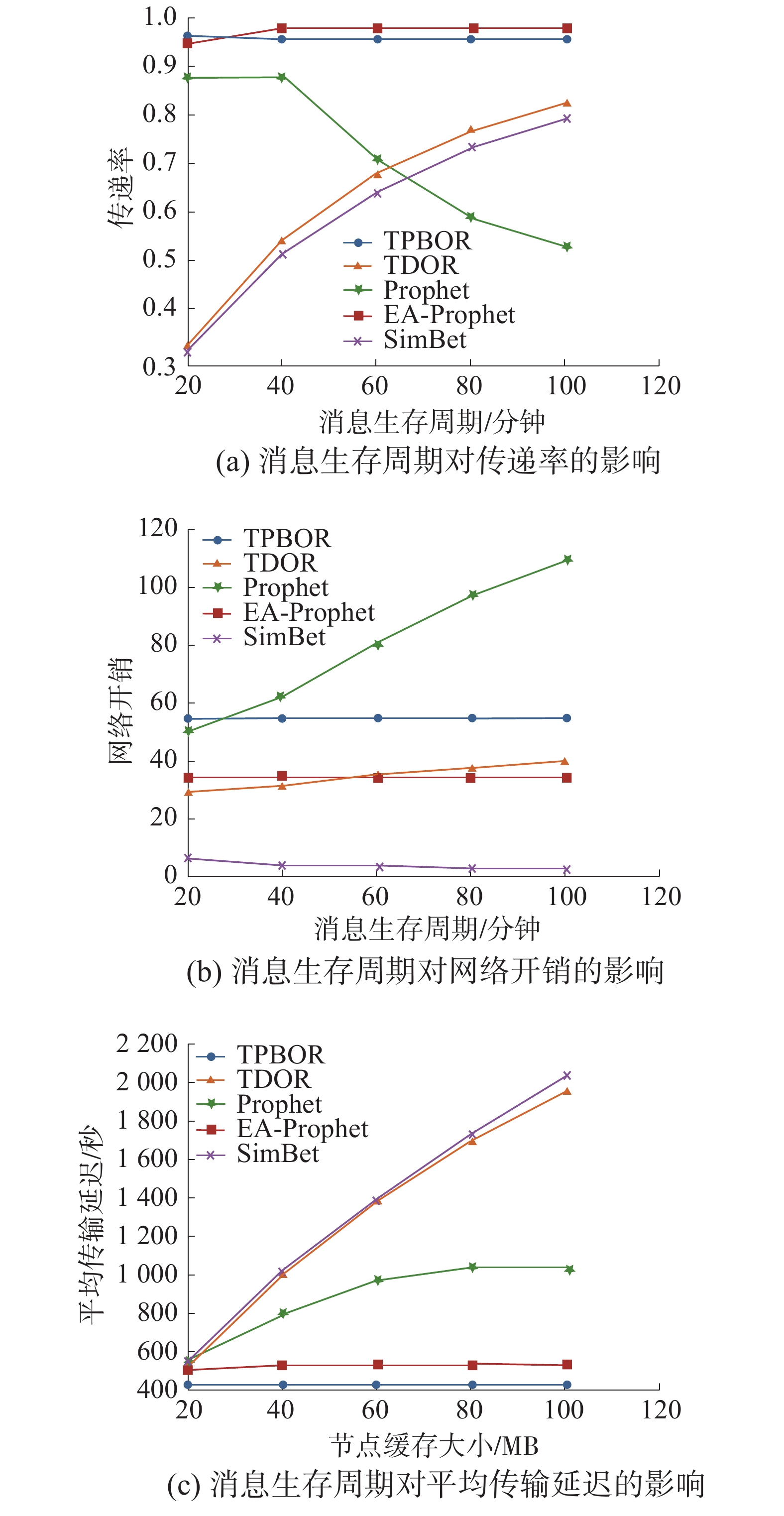Download: 图 9 消息生存周期对路由协议的影响

4 结论

  马华东, 袁培燕, 赵东. 移动机会网络路由问题研究进展[J]. 软件学报, 2015, 26(3): 600-616. (0)  DE RANGO F, AMELIO S, FAZIO P. Enhancements of epidemic routing in delay tolerant networks from an energy perspective[C]//Proceedings of the 2013 9th International Wireless Communications and Mobile Computing Conference, Sardinia, Italy, 2013: 731–735. (0)  HUANG Wei, ZHANG Sihai, ZHOU Wuyang. Spray and wait routing based on position prediction in opportunistic networks[C]//Proceedings of the 2011 3rd International Conference on Computer Research and Development, Shanghai, 2011: 232–236. (0)  DALY E M, HAAHR M. Social network analysis for routing in disconnected delay-tolerant MANETs[C]//Proceedings of the 8th ACM International Symposium on Mobile ad Hoc Networking and Computing. Montreal, Quebec, 2007: 32–40. (0)  NELSON S C, BAKHT M, KRAVETS R. Encounter-based routing in DTNs[C]//Proceedings of IEEE INFOCOM 2009, Rio de Janeiro, Brazil, 2009: 846–854. (0)  BISTA B B, RAWAT D B. EA-PRoPHET: an energy aware prophet-based routing PRoTOCOL for delay tolerant networks[C]//Proceedings of the 2017 IEEE 31st International Conference on Advanced Information Networking and Applications, Taipei, China, 2017: 670–677. (0)  WANG Tong, TANG Mengbo, SONG Houbing, et al. Opportunistic protocol based on social probability and resources efficiency for the intelligent and connected transportation system[J]. Computer networks, 2019, 149: 173-186. DOI:10.1016/j.comnet.2018.11.034 (0)  LINK J A B, SCHMITZ D, WEHRLE K. GeoDTN: geographic routing in disruption tolerant networks[C]//Proceedings of 2011 IEEE Global Telecommunications Conference, Houston, USA, 2011: 1–5. (0)  SOARES V N G J, RODRIGUES J J P C, FARAHMAND F. GeoSpray: a geographic routing protocol for vehicular delay-tolerant networks[J]. Information fusion, 2014, 15: 102-113. DOI:10.1016/j.inffus.2011.11.003 (0)  NICULESCU D, NATH B. Trajectory based forwarding and its applications[C]//Proceedings of the 9th Annual International Conference on Mobile Computing and Networking, San Diego, USA, 2003: 260–272. (0)  CAO Yue, KAIWARTYA O, ASLAM N, et al. A trajectory-driven opportunistic routing protocol for VCPS[J]. IEEE transactions on aerospace and electronic systems, 2018, 54(6): 2628-2642. DOI:10.1109/TAES.2018.2826201 (0)  陈思静, 张可. VANETs中的车辆移动规律性及轨迹预测研究[J]. 计算机工程与应用, 2016, 52(18): 139-143. DOI:10.3778/j.issn.1002-8331.1410-0313 (0)  SPAHO E, BAROLLI L, KOLICI V, et al. Performance evaluation of different routing protocols in a vehicular delay tolerant network[C]//Proceedings of 2015 10th International Conference on Broadband and Wireless Computing, Communication and Applications, Krakow, Poland, 2015: 157–162. (0)